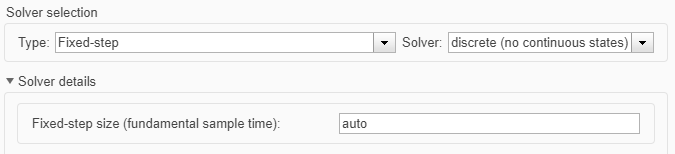Main Content

## Simulation Acceleration by Using GPU Coder

You can use GPU Coder™ to speed up the execution of your Simulink® model on NVIDIA® GPUs. GPU-accelerated computing follows a heterogeneous programming model. Highly parallelizable portions of the application are mapped into kernels that execute on thousands of GPU cores in parallel, while the remainder of the sequential code still runs on the CPU.

To perform GPU-accelerated simulation, model the compute intensive portions of your application in Simulink by using MATLAB Function (Simulink) blocks. When you simulate a model that contains a MATLAB Function block, the software partitions and generates CUDA® MATLAB® executable (MEX) code and integrates this code with the Simulink model.

The basic steps for simulation acceleration by using GPU Coder are:

• Create or open a model.

• Configure the model for GPU acceleration by selecting the Solver, Language, and other GPU-specific configuration parameters.

• Run the GPU accelerated model.

### Example: Sobel Edge Detection

The Sobel edge detection algorithm is a simple edge detection algorithm that performs a 2-D spatial gradient operation on a grayscale image. This algorithm emphasizes the high spatial frequency regions that correspond to the edges of the input image.

The Sobel edge algorithm computes the horizontal gradient (`H`) and the vertical gradient (`V`) of the input image by using two orthogonal filter kernels (`k` and `k'`). After the filtering operation, the algorithm computes the gradient magnitude and applies a threshold to find the regions of the images that are considered to be edges.

```k = single([1 2 1; 0 0 0; -1 -2 -1]); H = conv2(single(grayImage),k, 'same'); V = conv2(single(grayImage),k','same'); E = sqrt(H.*H + V.*V); edgeImage = uint8((E > threshold) * 255); ```### Create Edge Detection Model

1. Create a Simulink model and insert two MATLAB Function blocks from the User-Defined Functions library.

2. Add a Constant block and set its value to `0.4`.

3. Add a From Multimedia File block from the Computer Vision Toolbox™ library.

4. Open the Block Parameters dialog for the From Multimedia File block and set the File name parameter to `rhinos.avi`.

Set the Image signal parameter to ```One multidimensional signal```.

5. Add two Video Viewer blocks from the Computer Vision Toolbox library to the model.6. Double-click on one of the MATLAB Function blocks. A default function signature appears in the MATLAB Function Block Editor.

7. Define a function called `sobel`, which implements the Sobel edge detection algorithm. The function header declares `grayImage` and `threshold` as an argument to the `sobel` function, with `edgeImage` as the return value. Save Editor document to file.

```function edgeImage = sobel(grayImage,threshold) %#codegen % Define Kernel for Sobel edge detection k = single([1 2 1; 0 0 0; -1 -2 -1]); % Detect Edge H = conv2(single(grayImage),k, 'same'); V = conv2(single(grayImage),k','same'); E = sqrt(H.*H + V.*V); edgeImage = uint8((E > threshold) * 255); end ```

8. Open the block parameters for the MATLAB Function block. On the Code Generation tab, select ```Reusable function``` for Function packaging parameter.

If the Function packaging parameter is set to any other value, CUDA kernels may not get generated.

9. Modify the other MATLAB Function block to implement the RGB to grayscale conversion prior to the Sobel edge detection operation. Set the Function packaging parameter of the MATLAB Function block to `Reusable function`.

```function gray = RGB2gray(RGB) %#codegen % Convert color image to grey image gray = (0.2989 * double(RGB(:,:,1)) + ... 0.5870 * double(RGB(:,:,2)) + ... 0.1140 * double(RGB(:,:,3))); end```
10. Connect these blocks as shown in the diagram. Save the model as `edgeDetection.slx`.11. To test the model for errors, simulate the model in the Simulink Editor. On the toolstrip, click Run.

To see all video frames during simulation, disable the Simulation > Drop Frames to improve Performance option of the Video Viewer block.### Configure Model for GPU Acceleration

Model configuration parameters determine the acceleration method used during simulation.

1. Open the Configuration Parameters dialog box. Open the Solver pane. To compile your model for acceleration and generate CUDA code, configure the model to use a fixed-step solver. This table shows the solver configuration for this example.

ParameterSettingEffect on Generated Code
Type`Fixed-step`Maintains a constant (fixed) step size.
Solver`discrete (no continuous states)`Applies a fixed-step integration technique for computing the state derivative of the model.
Fixed-step size`auto`Simulink chooses the step size.2. On the Simulation Target pane, enable GPU acceleration parameter.

Note

The Language parameter is automatically set to `C++`.

3. GPU Coder specific options are now visible in the Simulation Target > GPU Acceleration pane. For the purposes of this example, you can use the default values for all the GPU-specific parameters.4. To save and close the Configuration Parameters dialog box, click OK .

You can also use the `set_param` (Simulink) function to configure the model parameters programmatically in the MATLAB command Window.

```set_param('edgeDetection','GPUAcceleration','on'); ```

### Build GPU Accelerated Model

To build and simulate the GPU accelerated model, select Run on the Simulation tab or use the following MATLAB command:

```sim('edgeDetection'); ```

The software first checks to see if CUDA code was previously compiled for the model. If code was created previously, the software runs the model. If code was not previously built, the software first generates and compiles the CUDA code, and then runs the model. The code generation tool places the generated code in a subfolder of the working folder called `slprj/_slprj/edgeDetection`.### Limitations

• GPU code generation for MATLAB Function blocks in Stateflow® charts is not supported.

• When GPU acceleration is enabled, the code generator does not support Import custom code for importing custom authored CUDA source files (*.cu). Instead, use `coder.ceval` inside the MATLAB Function block.

• The MATLAB Function block does not support all the data types from the MATLAB language. For supported data types, refer to the block documentation.

## Support

#### Generating CUDA Code from MATLAB: Accelerating Embedded Vision and Deep Learning Algorithms on GPUs

Download white paper